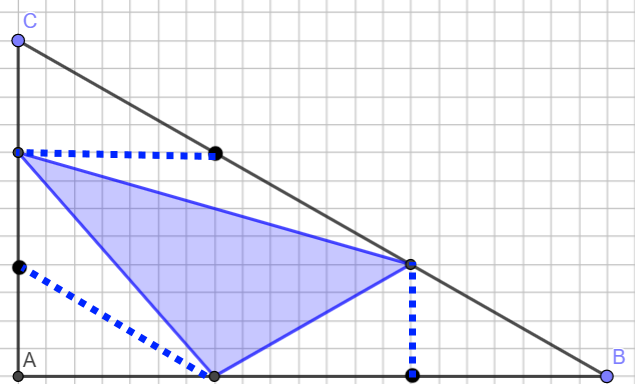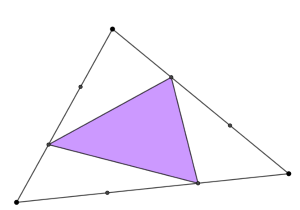#### You may also like### Fitting In

The largest square which fits into a circle is ABCD and EFGH is a square with G and H on the line CD and E and F on the circumference of the circle. Show that AB = 5EF. Similarly the largest equilateral triangle which fits into a circle is LMN and PQR is an equilateral triangle with P and Q on the line LM and R on the circumference of the circle. Show that LM = 3PQ### Look Before You Leap

Can you spot a cunning way to work out the missing length?### Triangle Midpoints

You are only given the three midpoints of the sides of a triangle. How can you construct the original triangle?

# Triangle in a Triangle

##### Age 14 to 16Challenge Level

Draw a right-angled triangle $ABC$, and mark a point $\frac13$ of the way along $AB$, $\frac13$ of the way along $BC$ and $\frac13$ of the way along $CA$.

Join your three points together to form a new triangle.
Can you work out the fraction of the original triangle that is covered by your new triangle?

You may wish to explore using the interactive diagram below.

Try a few examples. What do you notice?
Can you explain why?

Perhaps it might help to add some extra lines... click below to see.We started with a right-angled triangle... what about other triangles?What fraction of the triangle is shaded purple?
Can you prove it?

You may wish to try Areas and Ratios and Another Triangle in a Triangle next.